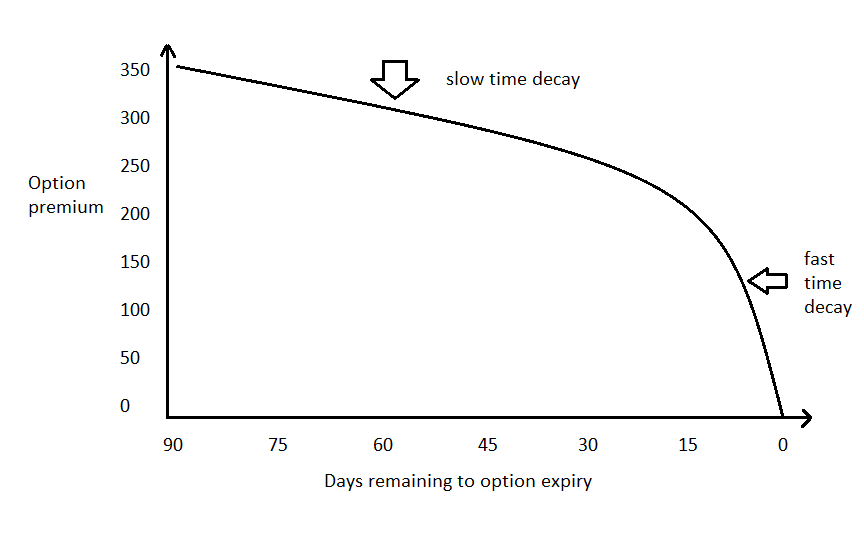# THETA EFFECT- BIGGEST RISK FOR OPTIONS BUYER“Time is money” we all know this adage.
If we have sufficient time to perform any task, whether it is competitive exam, whether we have to reach at any destination or any other then there is higher chance that we can do that.
Before we come to theta let’s take an example.

Suppose you are driving a car and you have to complete your journey of 100 km. if you have 24 hours to complete this task, then there is high probability that you will easily complete this task. But you have 5 hours then the probability will be moderate and if you have only 1 hour then probability will be very low, only one thing which can help you is your speed, otherwise one small tea break will take you in the trouble.

Now simply we are going to take it with option premium suppose we are choosing a strike price of 500 when stock is trading at 450 and we have complete 1 month. Probability of reaching at 500 will be very high because we have enough time. But if we have only 15 days then probability will be moderate and if have only 5 days then probability will be very low.

Suppose we have reached at 460 and 15 days passed, then it will a challenge to reach another 40 point in just 15 days. Like our car speed our underlying speed will also matter.

UNIVERSAL TRUTH:-Time value will be zero at the time of expiry and Theta is time value component.

Theta is not constant nor linearAs we can see in graph amount of decay indicated by theta tends to be gradual at first and accelerates as expiration approaches.
If stock does not have tendency to move very fast then we have to keep in mind that stock should have enough time to reach at the desired level.

#### IS THERE ANY WAY TO REDUCE THETA EFFECT?:-

Yes there are certain things through which we can reduce time decay effect.
Theta is responsible for time passing
Theta is enemy for buyer of option so GO FAR AS MUCH AS POSSIBLE
Theta is friend when selling an option.

#### THETA CORRELATION

Options (Calls and Puts) are always negatively correlated to the passage of time. They will decay

#### SUMMARY:-

The longer an options has until it expire, the less its price is affected by time passing. (that is why we buy only 90+days option & we sell only -60 days)
Theta only affects the time value portion of an option (that is why in order to lessen the exposure of time decay we use ITM option when buying them or in order to maximize the exposure of time decay we use OTM option when selling them)
OTM OPTION -> SELL OPTION